# NE555 tutorial: three useful circuits

### June 20, 2020 • tutorial

The NE555 is an extremely versatile integrated circuit that can be used for a wide variety of situations and projects. Today we will learn about what is inside an NE555, and how we can use it to build an oscillator, a timer, and a flip flop switch with a few capacitors, resistors, and of course the NE555.

## What's inside an NE555?

The NE555 is an integrated circuit, meaning that it combines a lot of components in a very small package. This package is called DIL8, which stands for dual-inline, and the 8 tell us that the NE555 has eight terminals. Here you can see the NE555 chip with its eight terminals: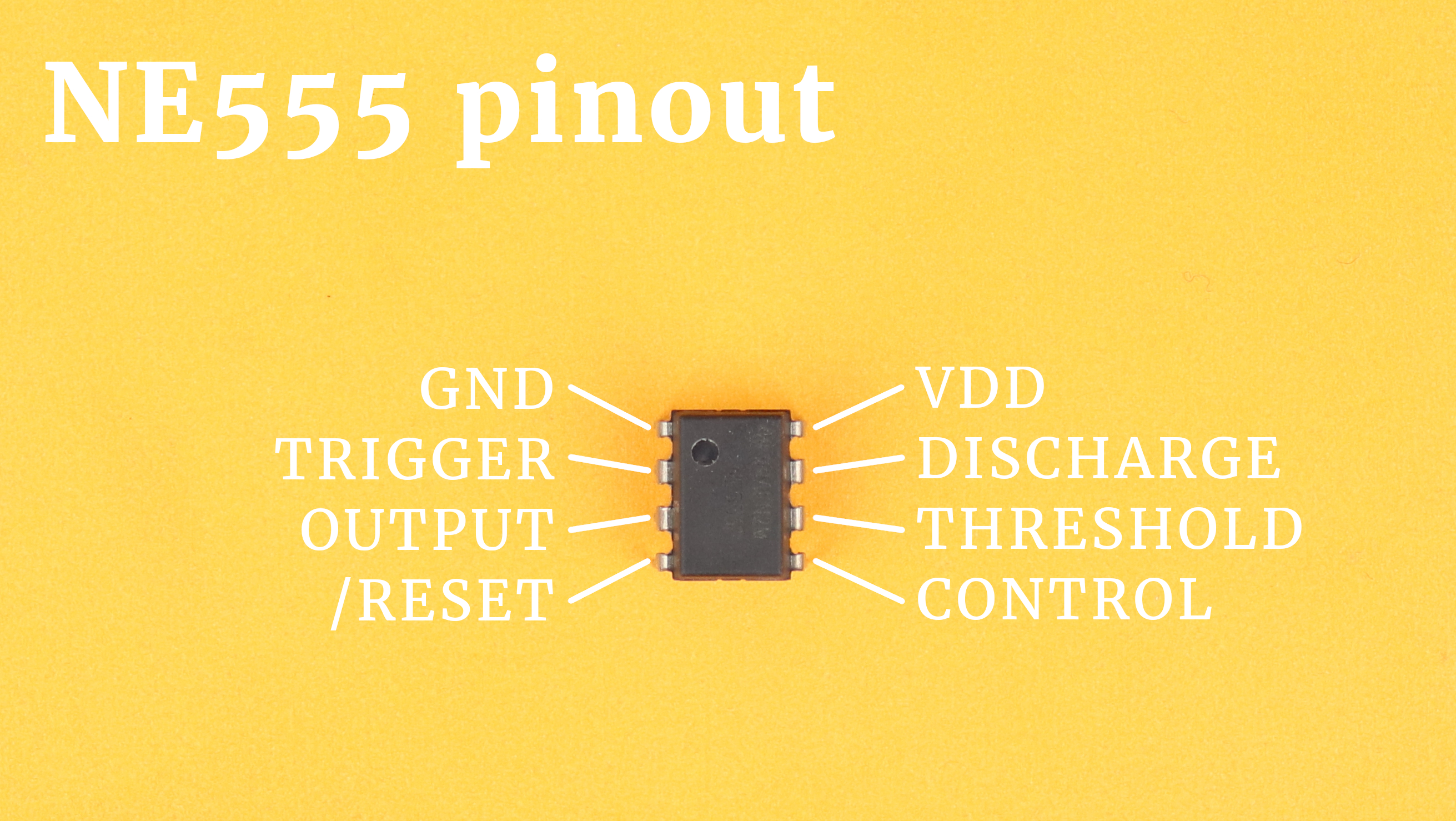To understand what each of these terminals do let us take a look inside:Let's go through step by step!

• VDD and GND are the connections for the positive and negative power supply terminals. Together with the resistors R1, R2, and R3 this generates a voltage divider into three equal parts. The potential between R1 and R2 is ⅔VDD and the potential between R2 and R3 is ⅓VDD. If you use a +9V power supply, which we will do later, then these two voltages are 6V and 3V. Some people say that the three 5kΩ resistors are what gives the NE555 its name, but apparently this has been a happy coincidence and was not done intentionally.
• COMP1 and COMP2 are comparators. They have two inputs, here called + and -, and one output. Whenever the voltage at + is larger than the voltage at -, they output VDD (a logical 1), and whenever the voltage at - is larger than the voltage at +, they output 0V (a logical 0).
• RS1 is a so-called RS flip flop. Its operation is very simple: whenever S is 1 and R is 0, the output Q is set to 1 as well. Whenever R is 1 and S is 0, the output is reset to 0. When both R and S are 0, nothing happens. When both R and S are 1, the output is undefined, so we should avoid that configuration. The additional reset input overrides anything that happens at the R and S inputs. When the reset input is low, the output Q gets reset to 0, and when reset is high, the output remains unchanged.
• INV1 is a simple inverter: if its input is 1 its output is a 0, and if its input is a 0 its output is a 1 :)
• T1 is an NPN transistor. It is activated whenever Q is 0, and it is deactivated whenever Q is 1. More on that below!

Okay, now we know what the components are we can talk about the external inputs. We already talked about the power connections VDD and GND, but what do the other pins do?

• CONTROL is a reference pin that we can use to change the voltage at the - input of COMP1: we could connect it to VDD with a different resistor and therefore change the voltage. Here we don't need it, so we mostly connect it to ground with a 10nF capacitor for stability.
• TRIGGER is the - input of COMP2. Whenever its voltage is less than ⅓VDD, the output of COMP1 goes high, and the RS flip flop is set so that Q is high, too. This “triggers” the output of the NE555 to go high as well, and that's where the name of this pin comes from.
• THRESHOLD is the + input of COMP1, and it can be used to turn the output of the NE555 off. How? If the voltage at this pin exceeds the control voltage, the output of COMP1 goes from 0 to 1, which resets the RS flip flop: then, its output Q is 0, and the output of the NE555 is zero, too. It gets its name from the fact that it resets the NE555 if the voltage surpasses a certain threshold (which is ⅔VDD in this case).
• OUTPUT spits out the value of the Q output of the RS flip flop. It can drive up to 200mA and can be directly connected to small loads. It is a push-pull output, meaning that it can drive loads both against VDD and against GND.
• DISCHARGE is a very helpful pin when using the NE555 as a timer. In the standard configuration, the discharge pin is connected to ground via the transistor T1. Why? Because in the default state the output of the RS flip flop is 0, which gets inverted by INV1 and therefore drives the base of T1. This in turn connects the discharge pin to ground. If the RS flip flop is set, however, the discharge pin is floating. This pin gets its name from that fact that we can use it to charge and discharge timing capacitors, and we will talk a lot about it below :)
• /RESET overrides the RS flip flop. It is an inverted pin, which is why we call it /RESET instead of RESET. This means that if /RESET is set to 1 the NE555 works in the normal configuration (which is why in many circuits the /RESET pin is tied to VDD permanently). If /RESET is connected to GND, however, it resets the flip flop and overrides anything going on on its R and S inputs. This pin is very useful when we want to use the NE555 as a bistable flip flop and whenever we don't need the timing features.

Now that we understand the basics, let's look at three very useful circuits based on the NE555: an oscillator (“astable mode”), a timer (“monostable mode”), and a simple flip flop (“bistable mode”). Don't worry when you still have some questions at this point, I know it's a bit abstract. I hope that after looking at some applications it will become much clearer. Let's go! :)

## NE555 as an oscillator (“astable” mode)

One of the most common uses of the NE555 is as an oscillator that provides a periodic ON/OFF signal that you can use to make an LED blink or use as a clock signal for digital circuits. It is also called “astable” because it does not have a stable state: it keeps switching between on and off! This is the schematic: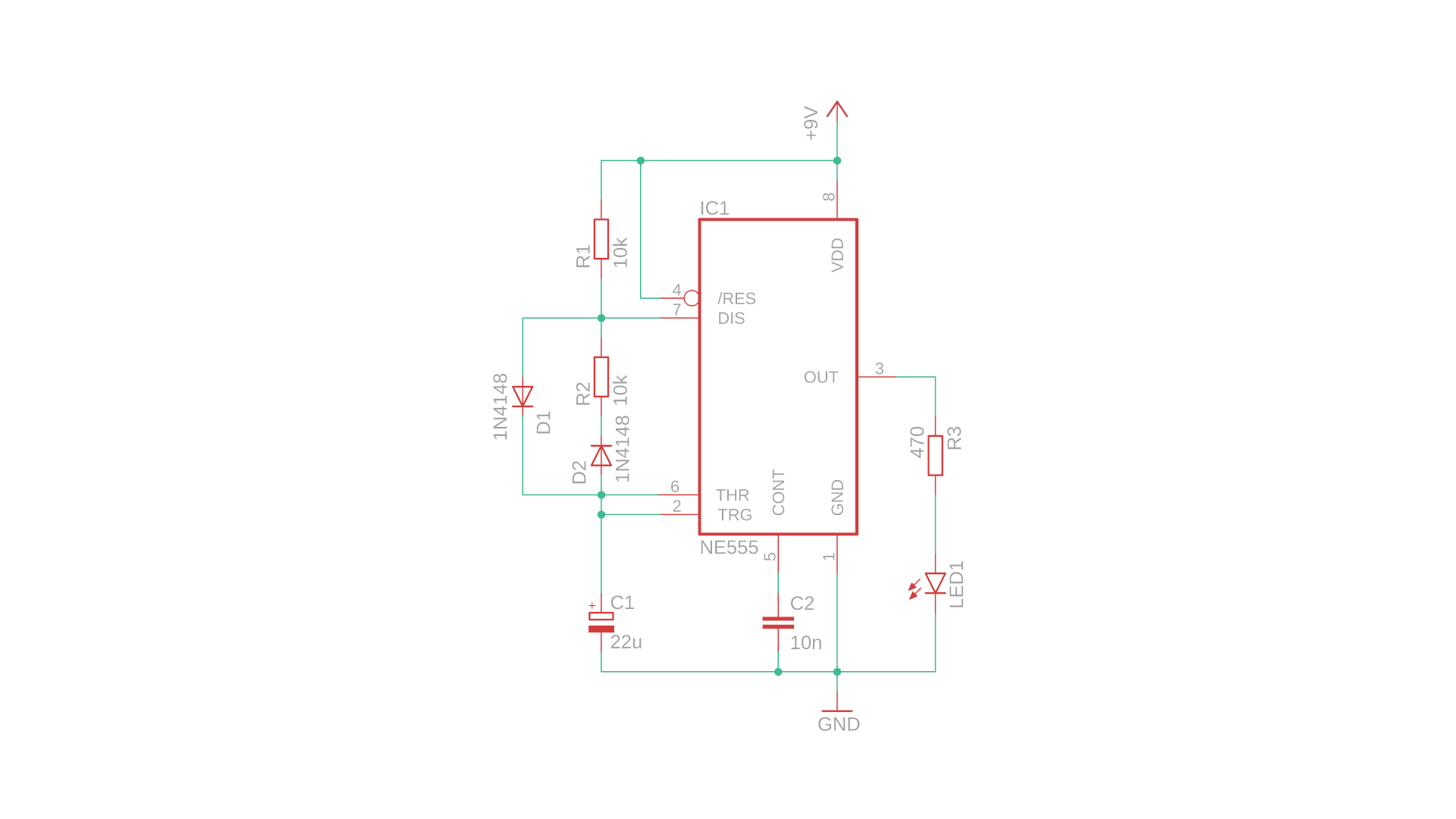Okay, let's take a closer look now and figure out how it all works:

• When the circuit is first powered on the capacitor C1 is uncharged, which means that the voltage at the TRIGGER pin is zero. This sets the internal flip flop and turns on the output, and disables the discharge transistor.
• The capacitor C1 is now charged through resistor R1 and diode D1, and the voltage at the TRIGGER pin soon exceeds ⅓VDD, but nothing happens yet. Only when the voltage at C1 exceeds ⅔VDD at the TRHESHOLD pin the internal flip flop is reset, turning the NE555's output off and enabling the discharge transistor.
• The capacitor C1 is now discharged through resistor R2 and diode D2, and the voltage at C1 decreases. If it falls below ⅓VDD, the TRIGGER pin will again set the NE555's internal flip flop to 1. The capacitor is no longer discharged and can therefore be charged through R1, and the loop begins all over again.
• The diodes D1 and D2 are there so that the capacitor charges only through R1 and only discharges through R2. This way, the on and off times can be adjusted independently, which can be very convenient.

LED1 blinks on and off, and the on-time and off-time are determined by the capacitor C1 as well as the resistors R1 and R2. You can calculate them like this:In our example, R1 = R2 = 10kΩ and C1 = 22μF. This means we need to substitute 10 and 22 in the formula, which gives us ton = toff = 152ms. This means the blinking frequency is around 6.5Hz.

Now that we understand how the circuit works, let's build it on a breadboard! Here is what you need: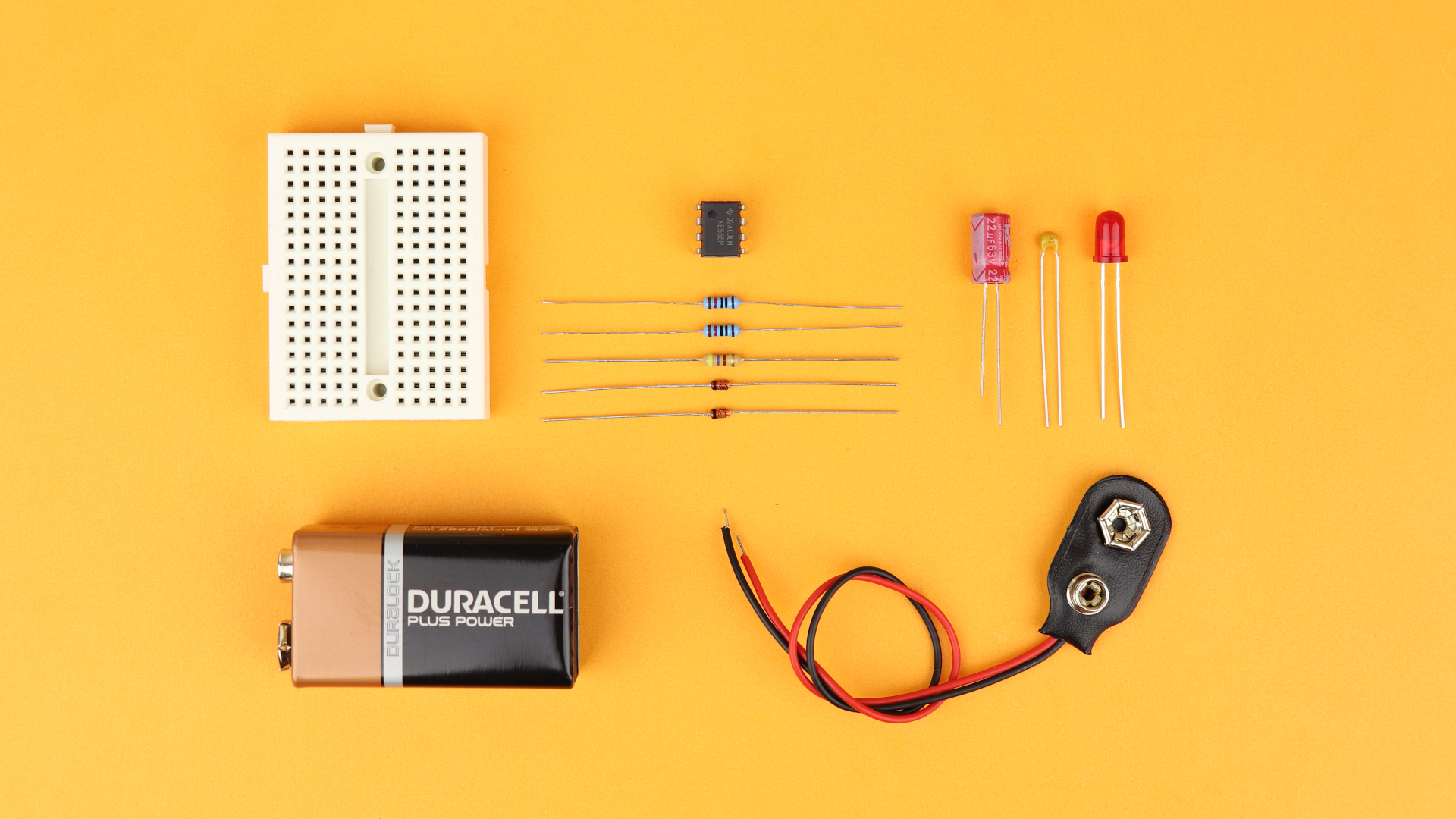You can find a detailed list of these components in the resources box. Let's build the circuit!

Here is the complete NE555 oscillator in all its glory. It's a bit difficult to capture the flashing LED in a photo, so be sure to check out the YouTube video as well if you want to see the oscillator in action :)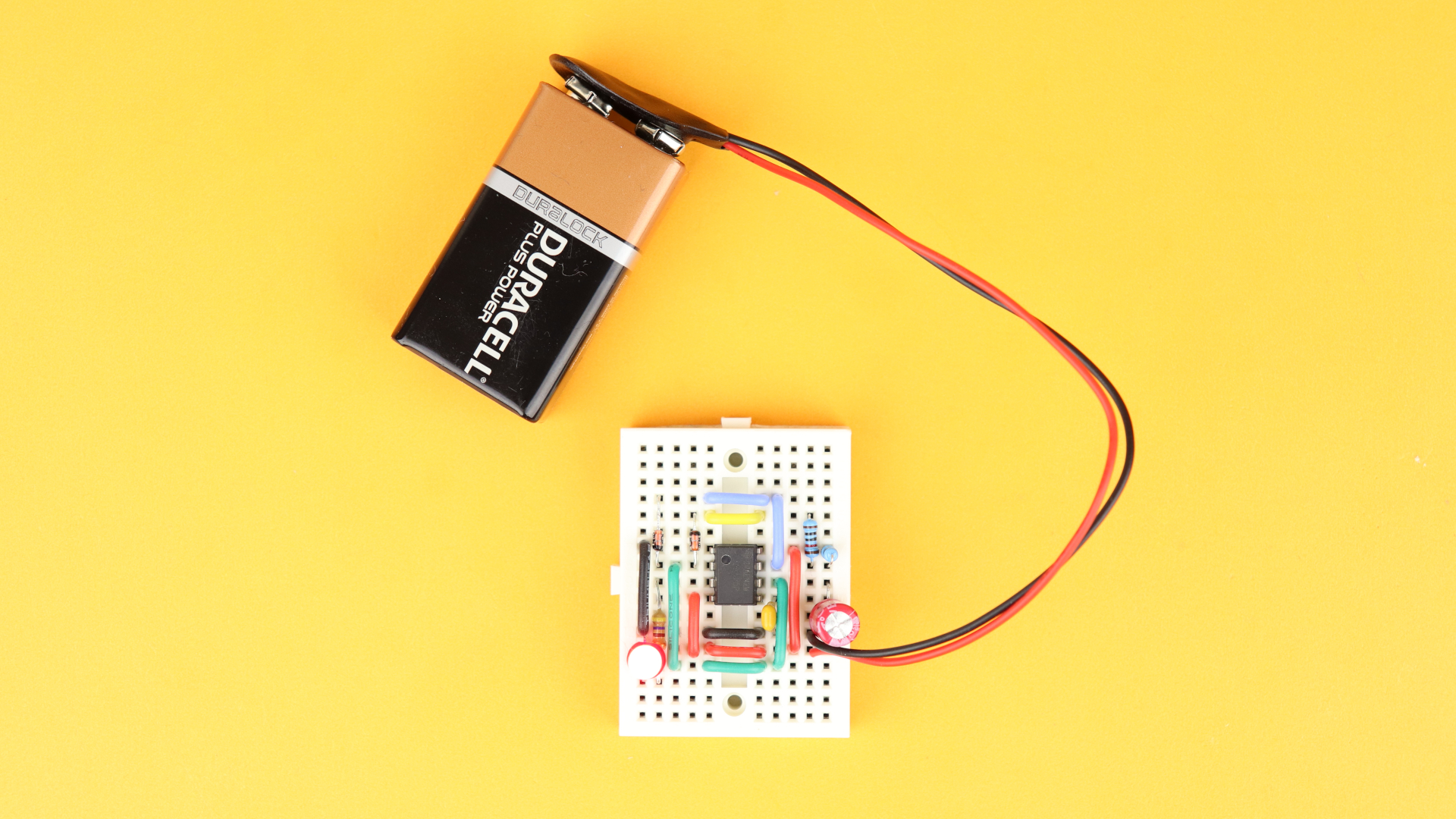You can also insert a potentiometer instead of the two resistors R1 and R2, and the circuit then looks like this:By turning the potentiometer you can adjust R1 and R2, and create different on and off times. In fact, this drive mode generates some sort of PWM because by turning the potentiometer you do not actually change the blinking frequency. Rather, you change the percentage that the LED is on.

If you want to use this as a realistic PWM to dim an LED (or to control a small motor), the capacitor C1 has to be chosen much lower in value so that the PWM frequency is high. If you choose R1 to be a 10kΩ potentiometer then C1=100nF would give you a PWM frequency of around 1kHz.

## NE555 as a timer (“monostable” mode)

Say you want to turn on an LED for 5 seconds, and then turn it off again automatically. This is exactly when you can use the NE555 as a timer! This mode is also called “monostable” because here the LED is always off (the stable state) unless you press a button and the LED gets turned on for a fixed amount of time.

Here is how the schematic looks like: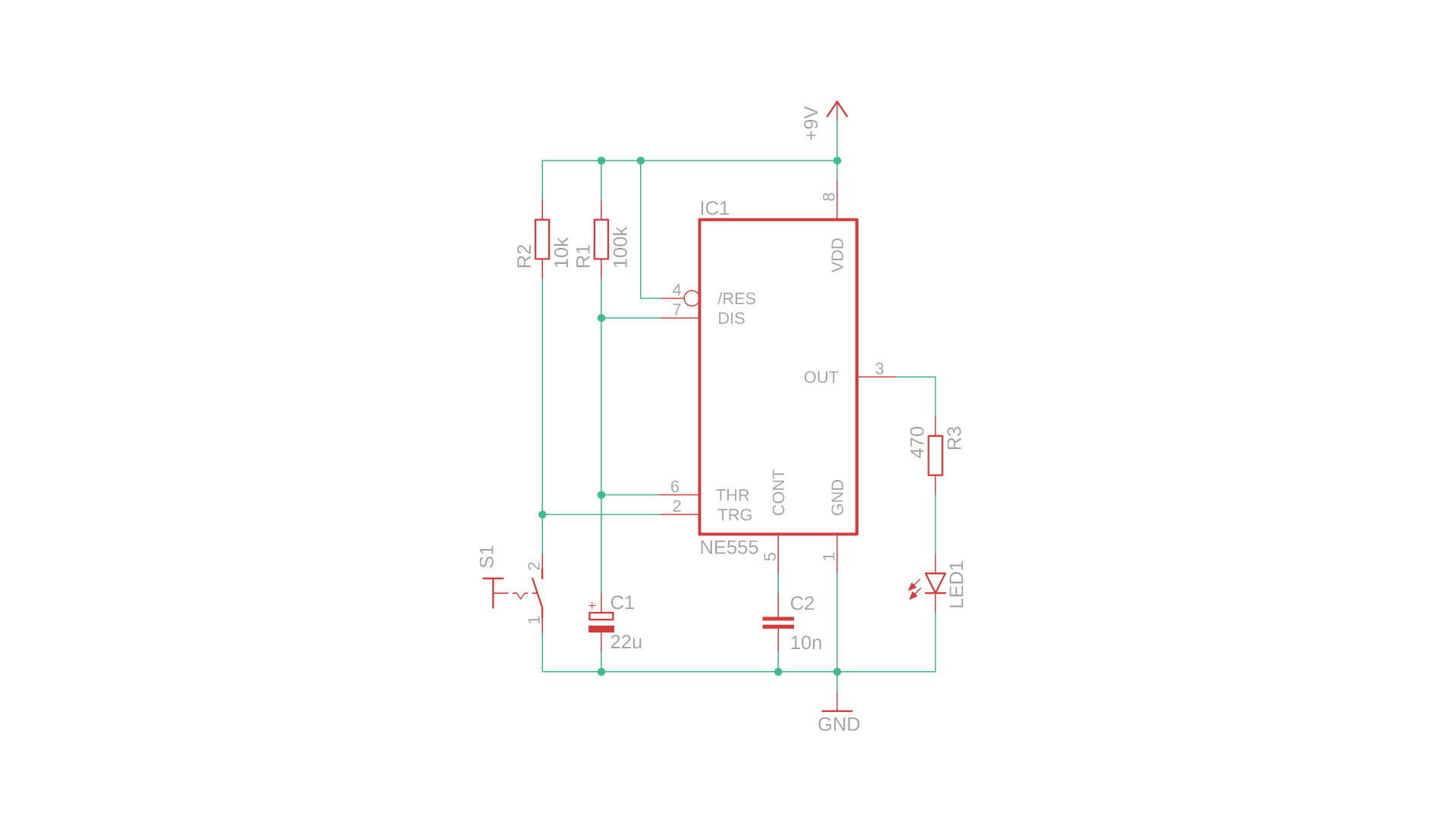Let's understand how it works!

• When nothing is going on and the circuit is powered on for the first time, the LED is off and the capacitor is discharged through the discharge pin because it is tied to ground.
• When you press the pushbutton S1, however, the trigger pin is grounded and sets the output of the NE555 to high. Also, it disables the discharge pin so that the capacitor C1 can now be charged over the resistor R1.
• The voltage on the capacitor slowly increases, and as soon as it reaches ⅔VDD the threshold pin resets the NE555 output to 0, and connects the discharge pin to ground, which immediately discharges the capacitor C1 (because there is no discharge resistor, this happens instantaneously without any delay).
• The capacitor C1 is now fully discharged, the output of the NE555 is turned off, and everything can begin all over again as soon as anybody presses the button S1.

We see: the LED is on only for a specified amount of time, and this time is determined by the capacitor C1 and the resistor R1 through which the capacitor is charged. This is the formula:In our example we have R1 = 100kΩ and C1 = 22μF, so we have to insert 100 and 22 in the above formula. This gives us a time of t = 2420 milliseconds or around 2.4 seconds. If you want to increase the time, you can just insert larger resistors or larger capacitors, and if you need a smaller time then insert a smaller resistor or a smaller capacitor :)

Okay, now that that makes some sense to us let's go ahead and build it on a breadboard! Here are the necessary electronic components: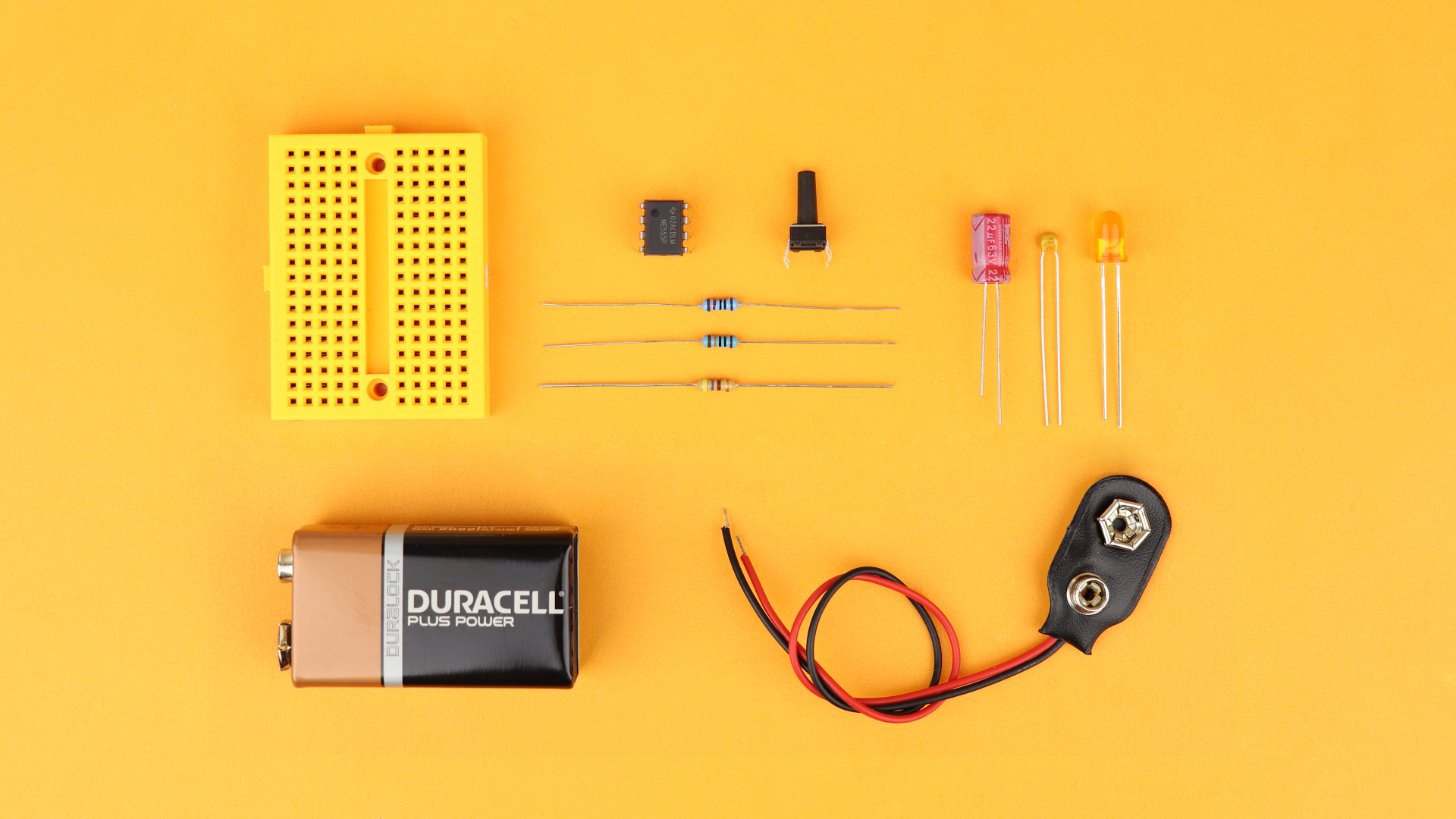Again, you can find a detailed list of these components in the resources box :) Let's build the circuit!

This is the finished NE555 timer, all set up and ready to go:And, just as expected, when you push the button the LED turns on for around 2.4 seconds, before turning off again. If you want to see this in action you can check out the YouTube video below :)

## NE555 as a flip flop (“bistable” mode)

At the very beginning we saw that the NE555 contains a flip flop, and we can forget about all the charging and discharging of capacitors and just use the flip flop on its own. This way, one pushbutton turns an LED on, and another pushbutton resets the LED back to zero. Both of these states are stable, which is why this mode is also called “bistable.” This is the schematic: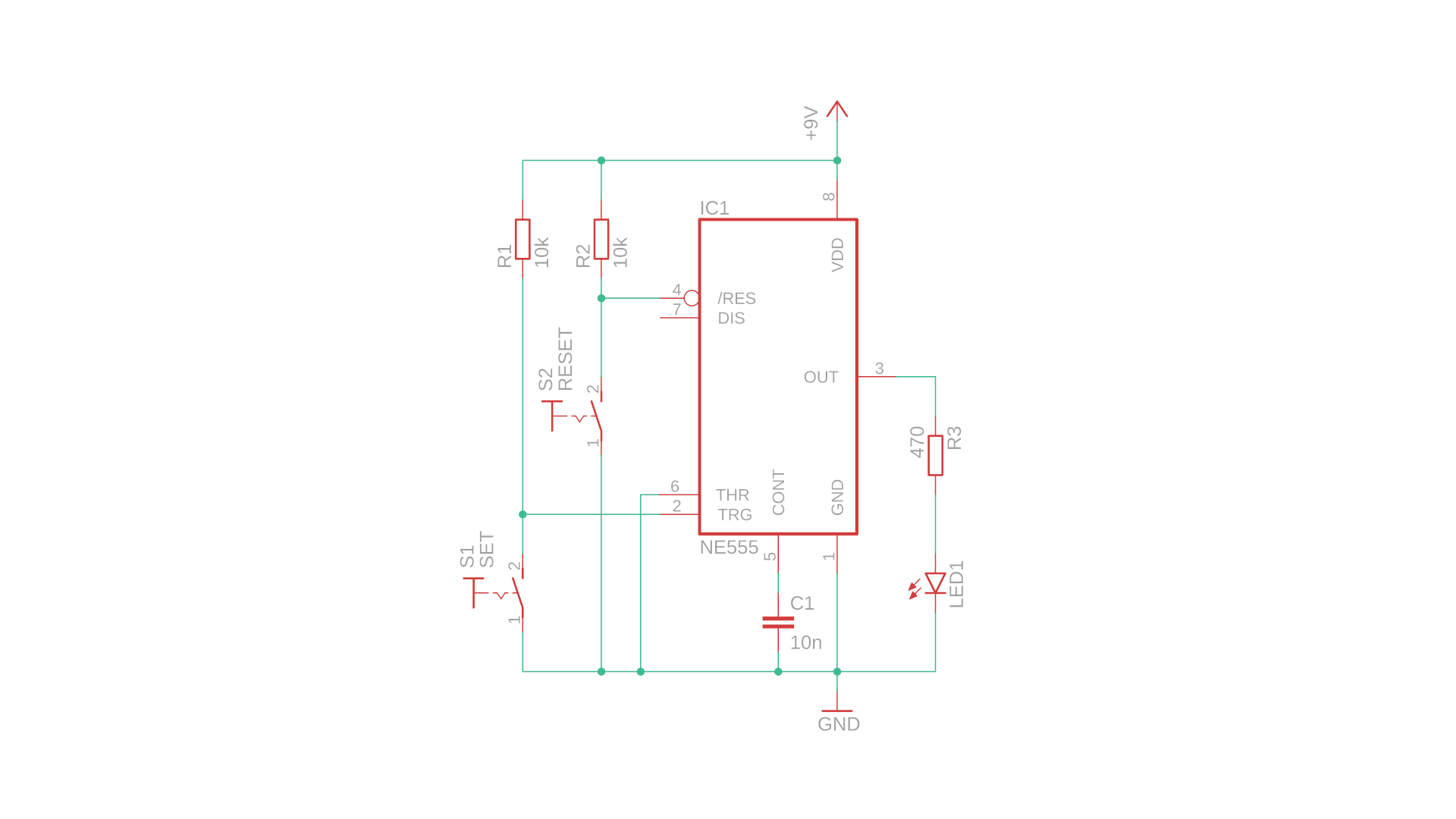It only uses the two pins TRIGGER and /RESET, and it works like this:

• When you first connect the circuit to power, trigger and /reset are both high. This means that the output of the NE555 is low and the LED is off. When you press reset (S2), nothing happens, and the output stays low.
• But when you press set (S1), then trigger is pulled to ground, which sets the output of the NE555 to high and the LED turns on. If you press S1 again, nothing else happens, the LED stays on.
• But if you now press reset (S2), the NE555 resets to its default state and the LED turns off again.

This is pretty simple, and there are not even any formulas this time because this mode is bistable. Here is what you need if you want to build this circuit on a breadboard: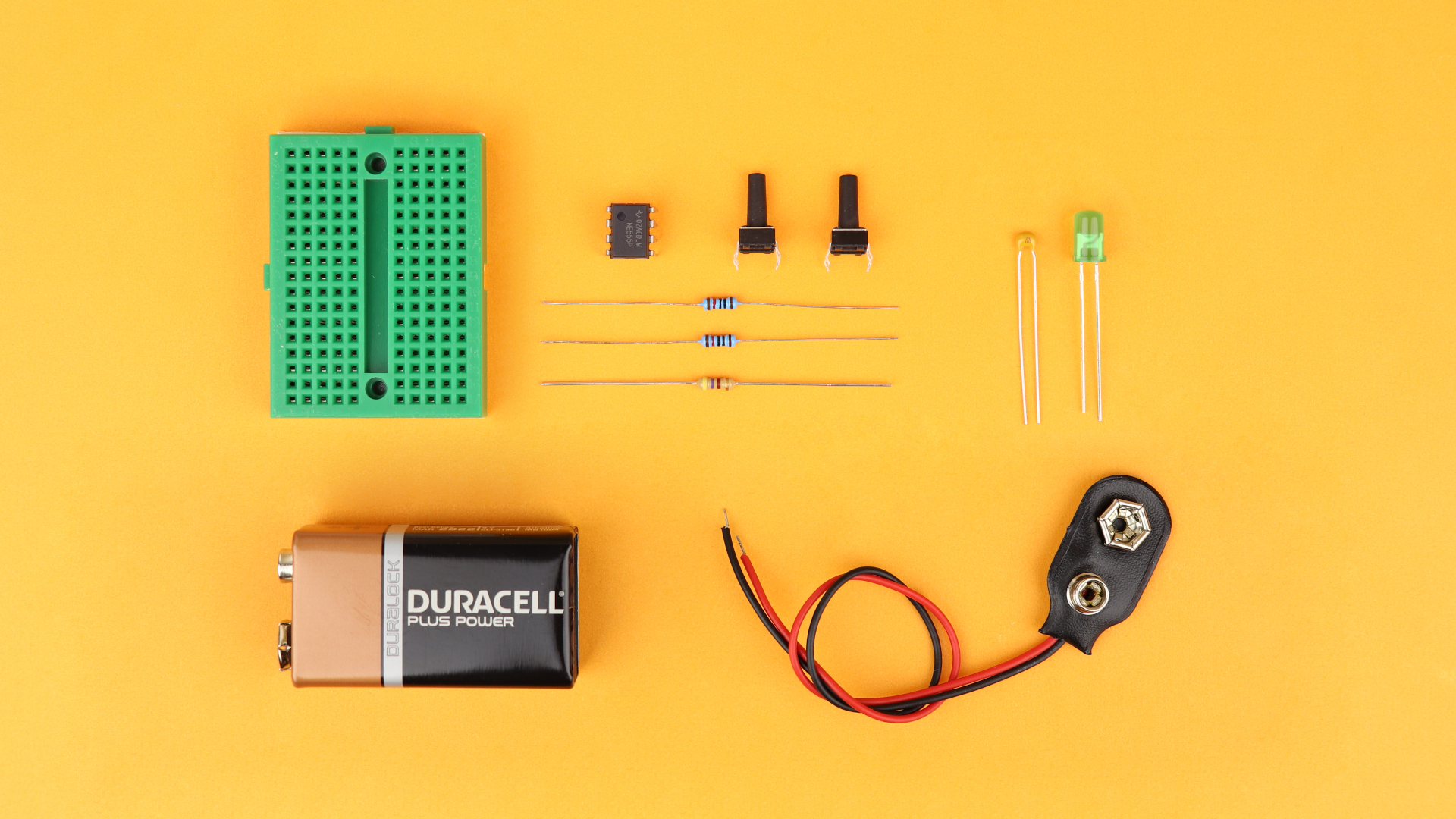As before, you can find a detailed list of these components in the resources box. Let's go and build it!

Here you can see the final circuit, after I already pressed the set button:I really like this circuit, it is very simple but extremely useful in many situations :)

I covered this entire tutorial in a dedicated YouTube video:

## Final thoughtsHere we are, you made it :) I hope I could show you that the NE555 is a very useful little integrated circuit, and I know that I will use it in a few upcoming projects. It's just a useful tool to have as a resource!

I also think that this tutorial shows that microcontrollers are not always necessary to do everything. Sometimes all you need is the good old NE555, and it has the additional advantage that you don't need a computer or any software to get it up and running.

Thank you for reading this article, and I hope you found it useful. If you have any questions, please get in touch on social media and I will do my best to get back to you. Have a great day!Beginner-friendly electronics tutorials and projects. Discover the joy of electronics! Keep reading.

## Oscillator: Components Needed

 1 × 170-pin breadboard (link) 1 × 9V block battery + battery clip 1 × NE555 timer IC (link) 2 × 10kΩ resistor (link, kit) 1 × 10kΩ potentiometer (link, kit) 2 × 1N4148 diode (link, kit) 1 × 22μF electrolytic capacitor (link, kit) 1 × 10nF ceramic capacitor (link, kit) 1 × LED of your choice (kit) 1 × 470Ω resistor (link, kit)

## Timer: Components Needed

 1 × 170-pin breadboard (link) 1 × 9V block battery + battery clip 1 × NE555 timer IC (link) 1 × 100kΩ resistor (link, kit) 1 × 10kΩ resistor (link, kit) 1 × 22μF electrolytic capacitor (link, kit) 1 × 10nF ceramic capacitor (link, kit) 1 × pushbutton (kit) 1 × LED of your choice (kit) 1 × 470Ω resistor (link, kit)

## Flip Flop: Components Needed

 1 × 170-pin breadboard (link) 1 × 9V block battery + battery clip 1 × NE555 timer IC (link) 2 × 10kΩ resistor (link, kit) 1 × 10nF ceramic capacitor (link, kit) 2 × pushbuttons (kit) 1 × LED of your choice (kit) 1 × 470Ω resistor (link, kit)

## Tools Needed

 1 × side cutter 1 × pliers

## Let's build a community

How did you get interested in electronics? What do you want to learn? Connect and share your story!

### Tag Cloud

• NE555
• oscillator
• timer
• flip flop
• astable mode
• monostable mode
• bistable mode
• beginner-friendly
• schematic
• tutorial